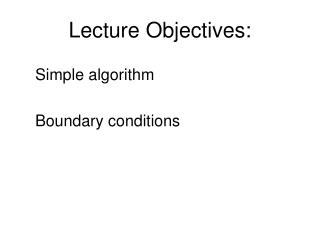Download PresentationLecture Objectives:# Lecture Objectives: - PowerPoint PPT Presentation

Download Presentation##### Lecture Objectives:

Download Policy: Content on the Website is provided to you AS IS for your information and personal use and may not be sold / licensed / shared on other websites without getting consent from its author. While downloading, if for some reason you are not able to download a presentation, the publisher may have deleted the file from their server.

- - - - - - - - - - - - - - - - - - - - - - - - - - - E N D - - - - - - - - - - - - - - - - - - - - - - - - - - -
##### Presentation Transcript

1. Lecture Objectives: Simple algorithm Boundary conditions

2. Navier Stokes Equations Continuity equation This velocities that constitute advection coefficients: F=rV Momentum x Momentum y Momentum z Pressure is in momentum equations which already has one unknown • In order to use linear equation solver we need to solve two problems: • find velocities that constitute in advection coefficients • 2) link pressure field with continuity equation

3. Pressure and velocities in NS equations How to find velocities that constitute advection coefficients? For the first step use Initial guess And for next iterative steps use the values from previous iteration

4. Pressure and velocities in NS equations How to link pressure field with continuity equation? SIMPLE (Semi-Implicit Method for Pressure-Linked Equations ) algorithm Dx Dx P E W Dx Ae Aw Aw=Ae=Aside We have two additional equations for y and x directions The momentum equations can be solved only when the pressure field is given or is somehow estimated. Use * for estimated pressure and the corresponding velocities

5. SIMPLE algorithm Guess pressure field: P*W, P*P, P*E, P*N , P*S, P*H, P*L 1) For this pressure field solve system of equations: x: ……………….. y: ……………….. z: Solution is: 2)The pressure and velocity correction P = P* + P’ P’ – pressure correction For all nodes E,W,N,S,… V = V* + V’ V’ – velocity correction Substitute P=P* + P’ into momentum equations (simplify equation) and obtain V’=f(P’) V = V* + f(P’) 3) Substitute V = V* + f(P’) into continuity equation solve P’ and then V 4) Solve T , k , e equations

6. SIMPLE algorithm start Guess p* p=p* Step1: solve V* from momentum equations Step2: introduce correction P’ and express V = V* + f(P’) Step3: substitute V into continuity equation solve P’ and then V Step4: Solve T , k , e equations no Converged (residual check) yes end

7. Other methods SIMPLER SIMPLEC variation of SIMPLE PISO COUPLED - use Jacobeans of nonlinear velocity functions to form linear matrix ( and avoid iteration )

8. Surface boundarieswall functions Wall surface Introduce velocity temperature and concentration Use wall functions to model the micro-flow in the vicinity of surface Using relatively large mesh (cell) size.

9. Surface boundarieswall functions Course mesh distribution in the vicinity of surface Y Wall surface Velocity in the first cell will depend on the distance y.

10. Surface boundary conditions and log-wall functions E is the integration constant and y* is a length scale Friction velocity u+=V/Vt y*=(n/Vt) y+=y/y* k- von Karman's constant The assumption of ‘constant shear stress’ is used here. Constants k = 0.41 and E = 8.43 fit well to a range of boundary layer flows. Surface cells Turbulent profile Laminar sub-layer

11. K-e turbulence modelin boundary layer Wall shear stress Eddy viscosity V Wall function for e Wall function for k

12. Modeling of Turbulent Viscosityin boundary layer forced convection natural convection

13. Temperature and concentration gradient in boundary layer Depend on velocity field • Temperature q=h(Ts-Tair) • Concentration F=hc(Cs-Cair/m) m=Dair/Ds m- segregation coefficient h = f(V) = f(k,e) Tair Ts Into source term of energy equation hC = f(V, material prop.) Cair Cs